﻿ Digital Circuits and Systems - Circuits i Sistemes Digitals (CSD) - EETAC - UPCBachelor's Degree in Telecommunications Systems and in Network Engineering## Synchronous data registers (plan Y) (plan C2)

n-bit parallel register: memory cell for n-bit words

1. Specifications

Design a 4-bit data register as a FSM based on plan Y (state signals defined as STD_LOGIC_VECTOR).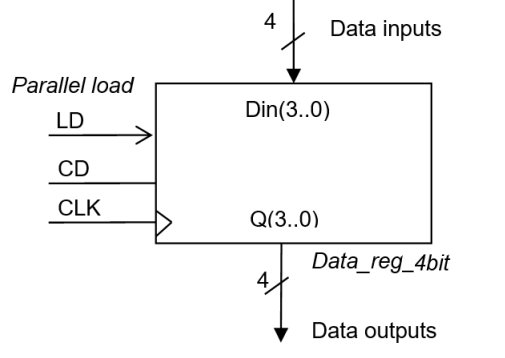Fig 1. Symbol of the Data_reg_4bit block and function table describing how the circuit works. When LD (parallel load) signal is sampled high, Din is saved as the data output Q.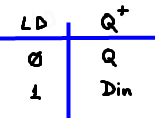Draw an example of timing diagram as in Fig. 2 to show how the circuit works. Be aware how sample control input LD or and Din vector have to be stable before and after CLK active edges (setup and hold times).Fig 2. Example of timing diagram showing when Din is sampled and when it is kept saved.

If we pay attention to the state diagram of such circuit as represented in Fig. 3, 16 values can be saved, thus it means a FSM up to 16 states. However, unlike counters, a large number of state transitions are possible (16 · 16 +16 = 272), from any state the register can jump to any other one. This architecture cannot be solved efficiently using plan X naming and labeling states and encoding them using any binary code as it was done in P6 applications or small counters such Counter_BCD_1digit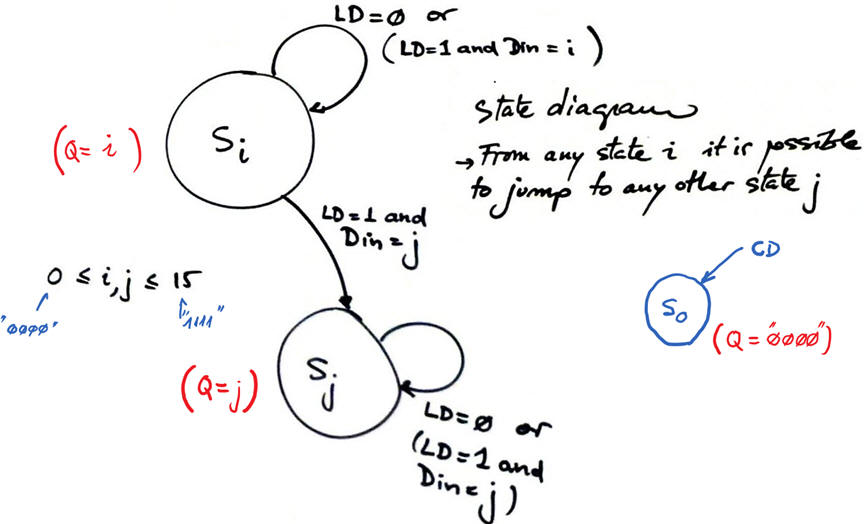Fig 3. State diagram.

2. Planning

Adapt the general architecture to this project.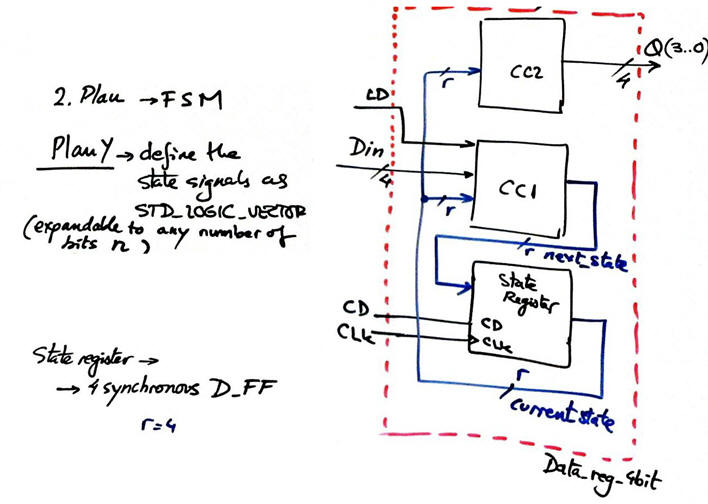Fig 3. FSM adaptation to the state register.

In Fig. 4 we see the structure of the FSM's state register. How many D_FF are used?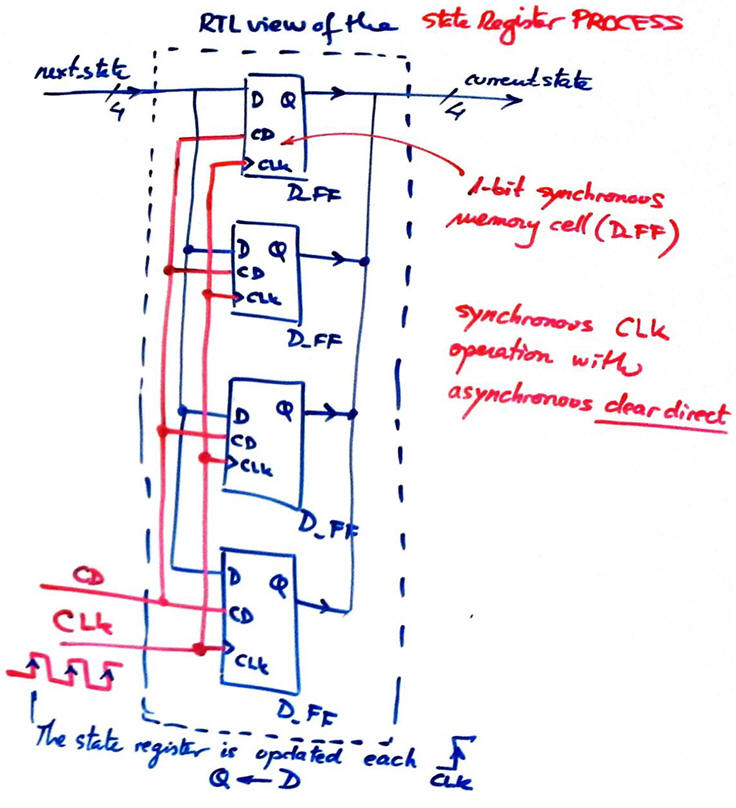Fig 4. State register consist of four D_FF working synchronously in parallel.

We can represent as well CC2 and CC2 truth tables and their equivalent as plan B flowcharts. Solving 272 states transitions is possible and simple to implement using STD_LOGIC_VECTOR signals as proposed in plan Y. Encoding FSM is solved in binary radix-2.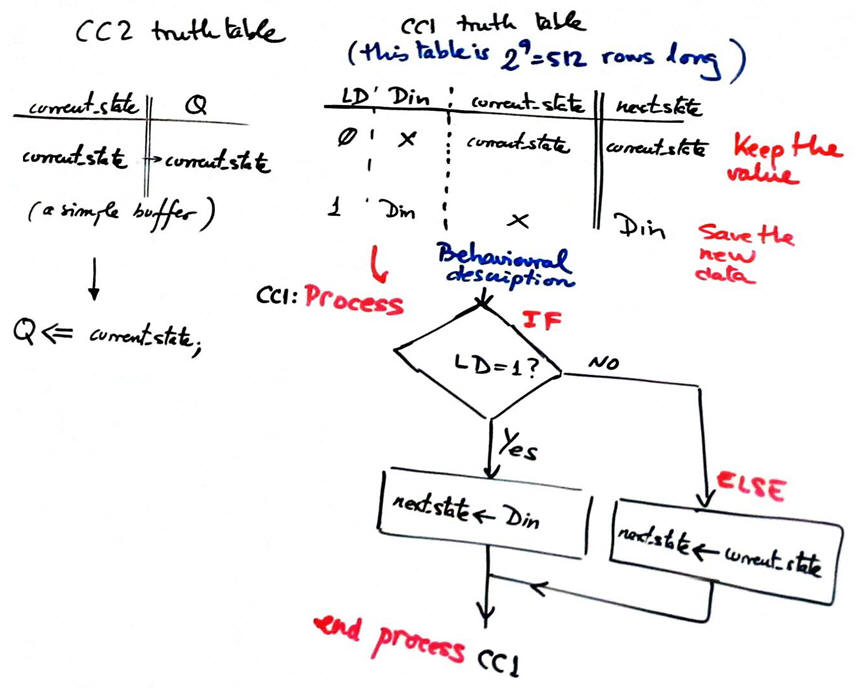Fig 5. CC1 and CC2 truth tables and flowcharts.

Project location:

C:\CSD\P7\Data_reg_4bit\(files)

Discussion on larger data registers, circuit expansion.

- How to invent Data_reg_nbit using plan Y? In the same way than the 4-bit data register, use n-size STD_LOGIC_VECTOR

- How to invent Data_reg_nbit using plan C2? Data_reg_4bit can be wired in parellel to reach any data size n. For instance a Data_reg_10bit is represented in Fig. 6.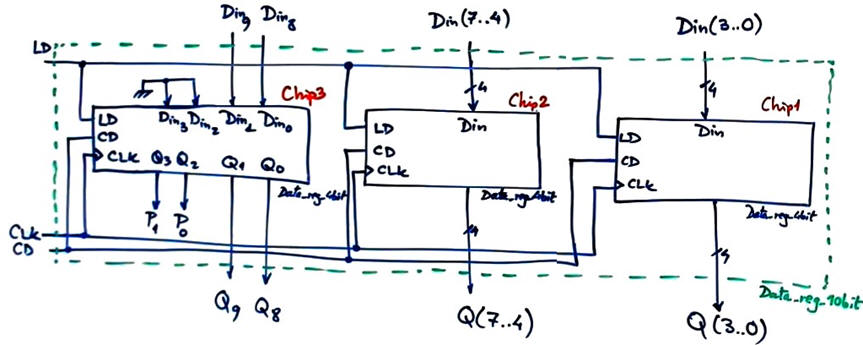Fig 6. Data_reg_10bit using plan C2 and components Data_reg_4bit.

- How to invent Data_reg_nbit using plan C2?

As shown in Fig. 7, universal counters such as Counter_mod16 forced to work always with CE = '0' or shift registers such as Shift_reg_4bit forced to work  with S(1) = S(0) = LD, can also be used as parallel data register.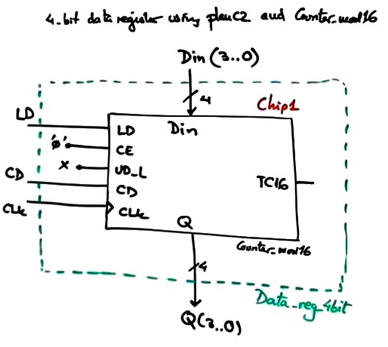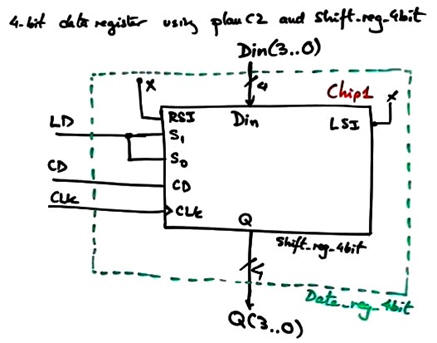Fig 7. Two alternative ways to implement a 4-bit data register using standard components.

3. Development

Translate the plan schematic in a single VHDL named Data_reg_4bit.vhd.

Start a synthesis project for a given CPLD or FPGA target chip.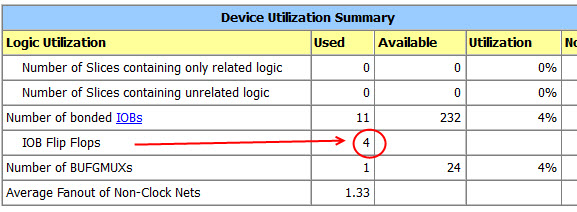Fig 8. The summary spreadsheet has to show the four D_FF used.

Print and discuss the RTL schematic.Fig 9. RTL view.

Print and comment the technology view. Fig. 10 shows the implementation in a Cyclone IV logic element. The D_FF already has an ENA (enable) additional input thus the LUT is simply used as a buffer for wiring input D.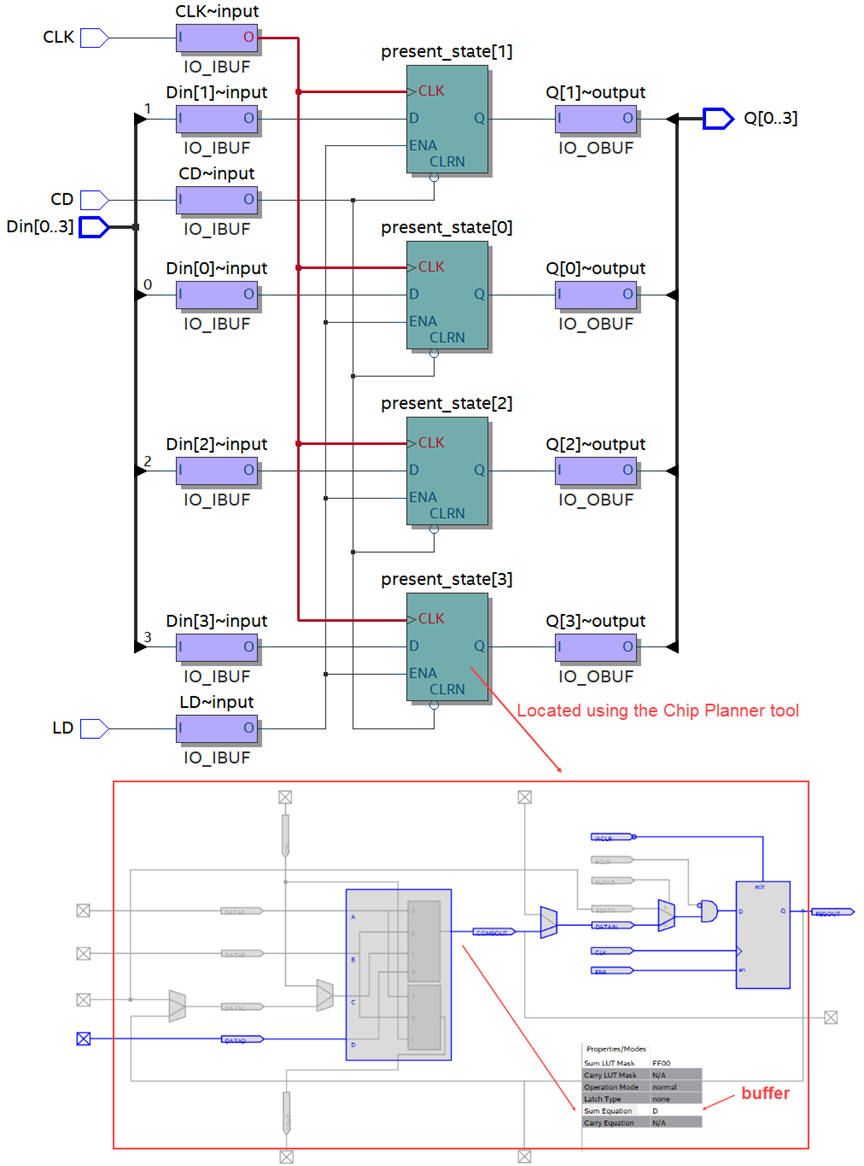Fig 10. Technology view.

4. Testing  (does the machine that we have invented work as expected?)

Draw the testbench fixture for testing the unit under test Data_reg_4bit and obtain its skeleton from Quartus Prime.Fig 11. Testbench fixture for running VHDL simulations.

Translate  the initial timing diagram sketch represented in Fig. 2 into a VHDL stimulus process for your testbench. Include as well constant CLK_Period. This example file can be used for copying constants and signal stimulus Data_reg_4bit_tb.vhd.

Run a ModelSim functional simulation and discuss results such in Fig. 12.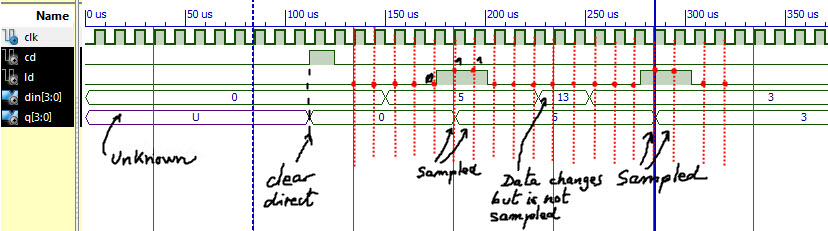Fig 12. Example of a timing diagram.

5. Testing  (gate-level, timing analyser)

Start a gate-level ModelSim functional simulation and measure propagation time from CLK to output tCO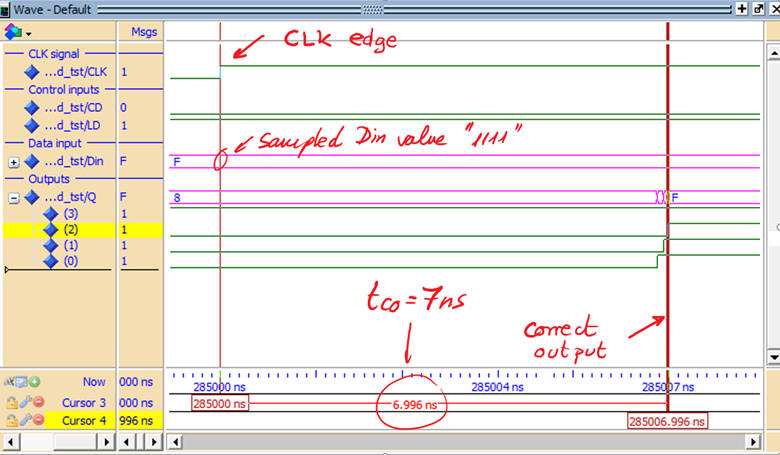Fig 13. Measuring tCO at a given active CLK edge transition.

Compare results with timer analyser tool.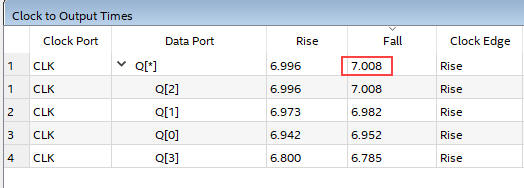Fig 14. Timing analyser spreadsheet calculating worst-case em>CO = 7 ns

How many times per second a 4-bit digital input can be sampled? fMAX < 1/tCO = 142.8 MHz

Can this Data_reg_4bit be used for sampling HI-FI audio signals at 44.1 kHz compact disk standard?

And as a front-end sampling circuit in a logic analyser instrument working at 100 MHz sampling ratio?

6. Report